## Mercator Projection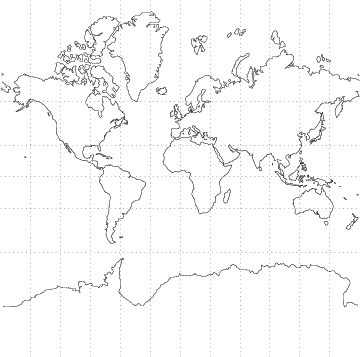The following equations place the x-Axis of the projection on the equator and the y-Axis at Longitude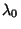, whereis the Longitude andis the Latitude.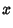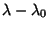(1)(2)(3)(4)(5)(6)

The inverse Formulas are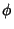(7)(8)

whereis the Gudermannian Function. Loxodromes are straight lines and Great Circles are curved.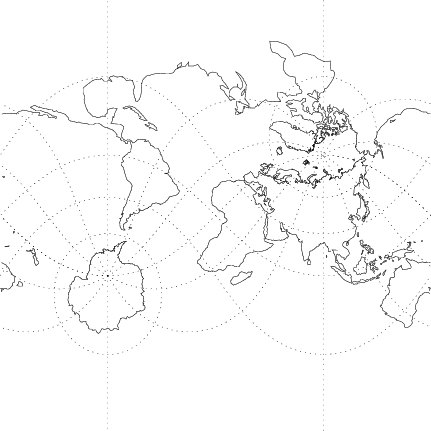An oblique form of the Mercator projection is illustrated above. It has equations(9)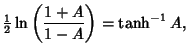(10)

where(11)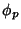(12)(13)

The inverse Formulas are(14)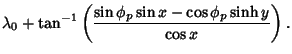(15)There is also a transverse form of the Mercator projection, illustrated above. It is given by the equations(16)(17)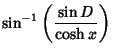(18)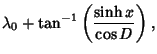(19)

where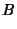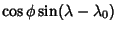(20)(21)

Finally, the universal transverse Mercator projection'' is a Map Projection which maps the Sphere into 60 zones of 6° each, with each zone mapped by a transverse Mercator projection with central Meridian in the center of the zone. The zones extend from 80° S to 84° N (Dana).

Dana, P. H. Map Projections.'' http://www.utexas.edu/depts/grg/gcraft/notes/mapproj/mapproj.html.